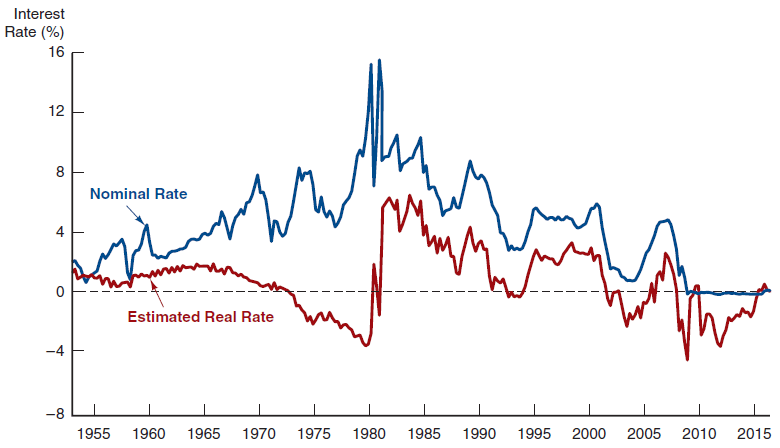# Real Interest Rate Calculator

Created by Tibor Pal, PhD candidate
Reviewed by Bogna Szyk
Last updated: Jan 16, 2022

If you would like to know how changes in the price level affect the real value of the interest you receive or pay, the real interest rate calculator will help you to compute and understand its concept. Below, you will find a simple way how to calculate the real interest rate, which is defined by the Fisher equation.

## What is the Fisher equation?

To find out the real interest rate, we need to adjust the nominal interest rate (the rates what you see in the newspaper or what a bank charges you for taking out a loan) by the changes in the price level (inflation or deflation), so it will reflect more accurately the true cost of borrowing. Thus, real interest rate shows you what it really costs borrowers to pay back their loans.

The concept of the real interest rate roots back to a canonical work of Irving Fisher (1930), one of the great monetary economists of the twentieth century, who formulated the relationship between interest rate and inflation. More precisely, the Fisher equation states that the nominal interest (i) rate equals the real interest (ir) rate plus the expected rate of inflation (πe).

i = ir + πe

After rearranging the variables, we find that the real interest rate equals the nominal interest rate minus the expected rate of inflation.

ir = i - πe

In case you don't know the nominal interest rate applied to your loans, you can determine it with our nominal interest rate calculator.

## How to apply the real interest rate formula?

To understand the logic behind the real interest rate, let's consider a case when you took a loan with a 6% interest rate (i = 6%), and you expect the price level to rise by 2% over the course of the year (πe = 2%). As a result, at the end of the year, you expect to pay back 4% in real terms, that is, in terms of real goods and services that money is worth at the end of the year.

ir = 6% - 2% = 4%

Therefore, your actual financial position as a borrower depends on the real interest rate:

• if the real interest rate is higher than zero, the amount you pay back is worth more in real terms than the money you borrowed.
• if the real interest rate is below zero, the amount you will pay back is less worth in real terms than the money you borrowed.

However, the situation is opposite when you lend or keep money in the bank. It follows that when the real interest rate is low in an economy, in general, there are more incentives to borrow and fewer incentives to lend.

## Importance of the real interest rate formula in economics

You can see on the graph that nominal and real interest rate in the United States often don't move in tandem. The gap between the nominal and real interest rate notably widened in the 1970s when the economy featured a rapid rise in the general price level. If we focus solely on the nominal interest rate, at the first glance it may seem that at this time it was expensive to borrow. However, because of the high inflation rate, the real interest rate was below zero; thus, the cost of borrowing was actually pretty low in real terms. So maybe now you are not surprised that during this time it became conventional wisdom to borrow, especially in the form of mortgage, as much as possible.Source: Mishkin, S.F. and Eakins, S.G. (2018). Financial Markets and Institutions, Global Edition (9e). Pearson Higher Ed USA

The course of real interest rate affects investments as well. Generally, higher nominal interest rates reduce investment, because higher rates increase the cost of borrowing and require investments to have a higher rate of return to be profitable. But now you know, what really matters is the real interest rate, so you may employ the real interest rate calculator before using other formulas, for example, the present value or future value calculators.

Tibor Pal, PhD candidate
Nominal interest rate
%
Expected inflation rate
%
Real interest rate
%
People also viewed…

### Boat loan

The boat loan calculator allows you to calculate the cost of taking out a boat loan.

### Books vs e-Books